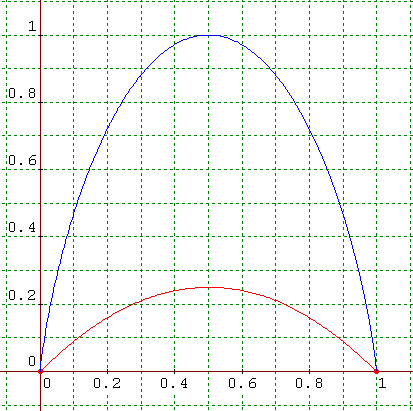# Help in proving this inequality

Can somebody help me please, I've tried solving this for hours but I still couldn't get it.

Given that a, b, c, d are positive integers and a+b=c+d.

Prove that if a∗b < c∗d,
then a∗log(a)+b∗log(b) > c∗log(c)+d∗log(d)

How do I do it?

RUber
Homework Helper
Start with the multiplication... You know that if ## A+B=C+D = N,the maximum for the product A*B or C*D is (N/2)^2.
So if AB < CD, then C and D are more central...which gives you A < C ≤ D < B.
From there use the fact that the log function is concave down.

Please post a little bit more about what you have tried, and where you are stuck.

The closest I've got is I've tried to log both sides of the 1st inequality giving log(a)+log(b) < log(c)+log(d) then I tried to make one side similar the the 2nd inequality but then I realized that I'm going in circles.

How do I use the concave down point?

Given a new constrant that A+B = C+D = 1
Does showing that:
d[ -1(a*log(a)+(1-a)*log(1-a)) ] / d[a] * d[ a*(1-a) ] / d[a] to be always greater than or equal to zero prove the original claim?

Since satisfying this means that the two functions grow and shrink together (albeit not in the exact amount).
y=-1/log(2)*(x*log(x)+(1-x)*log(1-x)) {[0,1]} // the a*log(a)+b*log(b)
y=x*(1-x) {[0,1]} // the a*b